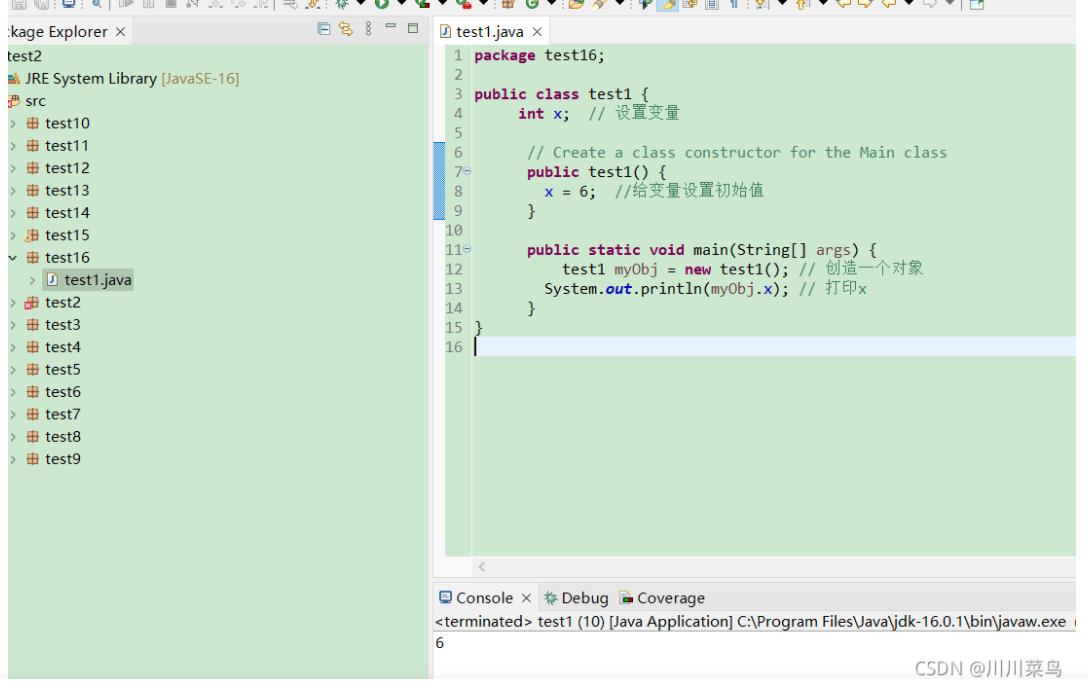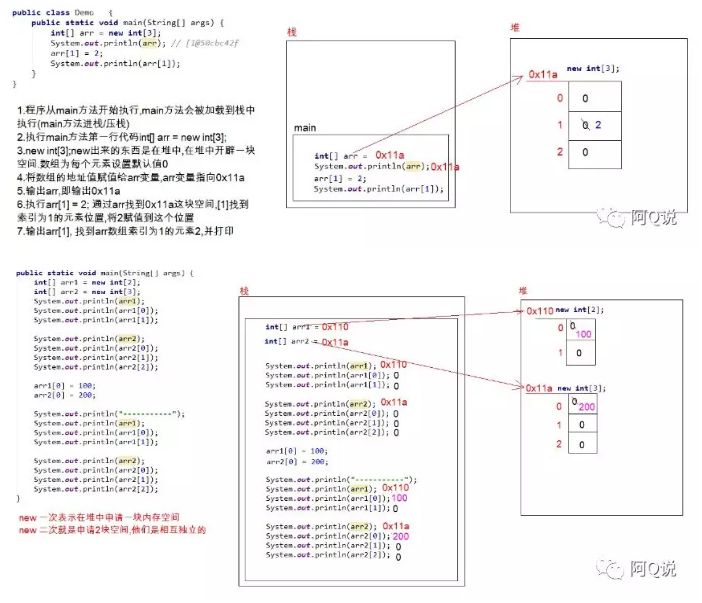# Java：函数，类，数组之间的运用

+关注继续查看

public class Demoe {
//计算一个数字因子的个数
public static int getLenght(int n){
int count = 0 ;
for(int i = 1 ; i <= n / 2 ; i++)
{
if(n % i == 0)
count++ ;
}
count++;
return count ;
}
//求一个数的因子(包含1也包含自己)，放入数组并返回
public static int[] getSons(int n){
int []a = new int[getLenght(n)] ;
int j = 0 ;
for(int i = 1 ; i <= n / 2 ; i++){
if(n % i == 0)
a[j++] = i ;
}
a[j] = n ;//自己也要放进去
return a ;
}
//获取两个正int类型数组中相同且最大的那个元素
public static int getTheSameMax(int []a , int [] b){

int max = 1 ; //正整数最小就是1
for(int i = 0 ; i < a.length ; i++){
for(int j = 0 ; j < b.length ; j++){
if(a[i] == b[j]){
if(max < a[i])
max = b[i] ;
break ;
}
}
}
return max ;
}

//打印数组元素
public static void PrintArray(int []a){
for(int i = 0 ; i < a.length ; i++)
System.out.println(a[i]+" ");
System.out.println();
}
}


public class HelloWorld {
public static void main(String[] args){
//	Scanner s = new Scanner(System.in);
//	System.out.println("请输入一个数字");
//	int num = s.nextInt();
//数组与函数的结合
print_arr(12,18);
}
//求两个数字的最大公约数和最小公倍数(辗转相除法)
//a.分别找出两个数的公约数，并放入数组
//b.找出最大的，就是最大公约数
//c.两数想乘/最大公约数 = 最小公倍数
public static void print_arr(int a , int b){
//得到a的公约数
int [] x1 = Demoe.getSons(a);
Demoe.PrintArray(x1);
//得到b的公约数
int [] x2 = Demoe.getSons(b);
Demoe.PrintArray(x2);
//找出相同且最大的数是最大公约数
int max = Demoe.getTheSameMax(x1, x2);
//最小公倍数
int min = a * b /max ;
System.out.println(a+","+b+"的最大公约数是"+"最小公倍数是"+min);
}

}Java面向对象高级--继承的应用----整形数组类、排序类、反转类
<p><img src="http://img.blog.csdn.net/20130924111658296" alt=""></p> <p>分析：</p> <p><img src="http://img.blog.csdn.net/20130924111727328" alt=""><br></p> <p></p> <pre name="code" class="java">c
1047 0Java中函数的递归调用

724 0Java：函数，类，数组之间的运用

662 0Java:函数
import java.util.Scanner; public class HelloWorld { public static void main(String[] args){ Scanner s = new Scanner(System.
686 0Java构造函数
Java构造函数
18 0Java 8 Predicate 函数接口
Java 8 Predicate 函数接口
18 016866 024786 0Java基础——函数与数组

21 0JAVA数组Java StringBuffer 和 StringBuilder 类

885 0
morixinguan
ITGEGE在线教育嵌入式开发讲师。 CSDN博客专家、CSDN-Linux特邀编辑、CSDN博乐、CSDN学院讲师，目前从事嵌入式开发领域，从事与单片机，Linux，android相关的产品开发。
499

1

OceanBase 入门到实战教程